## Fractals and the Fractal Dimension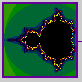### Mandelbrot and Nature

"Clouds are not spheres, mountains are not cones, coastlines are not circles, and bark is not smooth, nor does lightning travel in a straight line."(Mandelbrot, 1983).

### The Concept of Dimension

So far we have used "dimension" in two senses:

• The three dimensions of Euclidean space (D=1,2,3)
• The number of variables in a dynamic system

Fractals, which are irregular geometric objects, require a third meaning:

#### The Hausdorff Dimension

If we take an object residing in Euclidean dimension D and reduce its linear size by 1/r in each spatial direction, its measure (length, area, or volume) would increase to N=rD times the original. This is pictured in the next figure.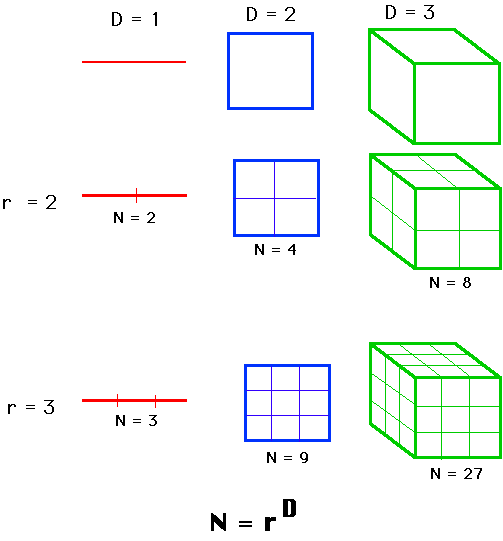We consider N=rD, take the log of both sides, and get log(N) = D log(r). If we solve for D. D = log(N)/log(r) The point: examined this way, D need not be an integer, as it is in Euclidean geometry. It could be a fraction, as it is in fractal geometry. This generalized treatment of dimension is named after the German mathematician, Felix Hausdorff. It has proved useful for describing natural objects and for evaluating trajectories of dynamic systems.

### The length of a coastline

Mandelbrot began his treatise on fractal geometry by considering the question: "How long is the coast of Britain?" The coastline is irregular, so a measure with a straight ruler, as in the next figure, provides an estimate. The estimated length, L, equals the length of the ruler, s, multiplied by the N, the number of such rulers needed to cover the measured object. In the next figure we measure a part of the coastline twice, the ruler on the right is half that used on the left.Measuring the length of a coastline using rulers of varying lengths.

But the estimate on the right is longer. If the the scale on the left is one, we have six units, but halving the unit gives us 15 rulers (L=7.5), not 12 (L=6). If we halved the scale again, we would get a similar result, a longer estimate of L. In general, as the ruler gets diminishingly small, the length gets infinitely large. The concept of length, begins to make little sense.

### The "Richardson Effect"

Lewis Fry Richardson first noted the regularity between the length of national boundaries and scale size. As shown next, the relation between length estimate and length of scale is linear on a log-log plot.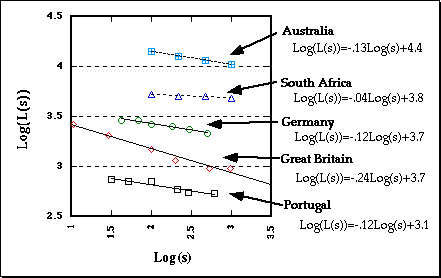The Richardson Effect.

Mandelbrot assigned the term (1-D) to the slope, so the functions are:
log[L(s)] = (1-D)log(s) + b where D is the Fractal Dimension.
For Great Britain, 1 - D = -.24, approximately. D = 1-(-.24) = 1.24, a fractional value.The coastline of South Africa is very smooth, virtually an arc of a circle. The slope estimated above is very near zero. D = 1-0 = 1. This makes sense because the coastline is very nearly a regular Euclidean object, a line, which has dimensionality of one. In general, the "rougher' the line, the steeper the slope, the larger the fractal dimension.

### Examples of geometric objects with non-integer dimensions

#### Koch Curve

We begin with a straight line of length 1, called the initiator. We then remove the middle third of the line, and replace it with two lines that each have the same length (1/3) as the remaining lines on each side. This new form is called the generator, because it specifies a rule that is used to generate a new form.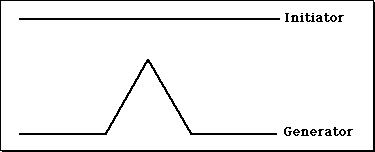The Initiator and Generator for constructing the Koch Curve.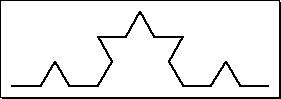The rule says to take each line and replace it with four lines, each one-third the length of the original.

Level 2 in the construction of the Koch Curve.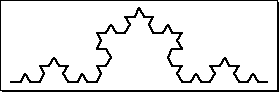Level 3 in the construction of the Koch Curve.

We do this iteratively ... without end.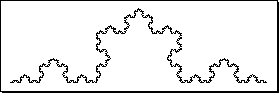The Koch Curve.

What is the length of the Koch curve?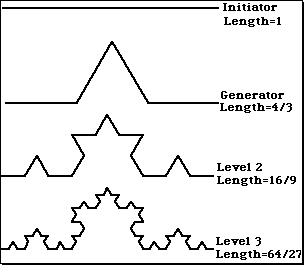The length of the curve increases with each iteration. It has infinite length. But if we treat the Koch curve as we did the coastline, ...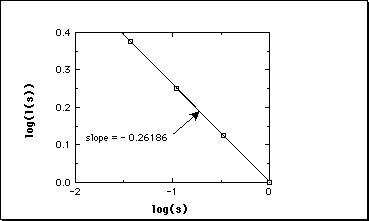The relation between log(L(s)) and log(s) for the Koch curve ...

we find its fractal dimension to be 1.26. The same result obtained from D = log(N)/log(r) D = log(4)/log(3) = 1.26.

#### Cantor Dust

Iteratively removing the middle third of an initiating straight line, as in the Koch curve, ...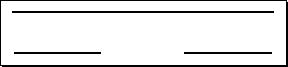Initiator and Generator for constructing Cantor Dust. ...

this time without replacing the gap...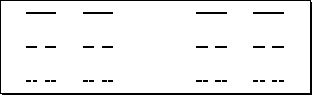Levels 2, 3, and 4 in the construction of Cantor Dust.

Calculating the dimension ... D = log(N)/log(r) D = log(2)/log(3) = .63 We have an object with dimensionality less than one, between a point (dimensionality of zero and a line (dimensionality 1).

#### Sierpinski Triangle

We start with an equilateral triangle, connect the mid-points of the three sides and remove the resulting inner triangle.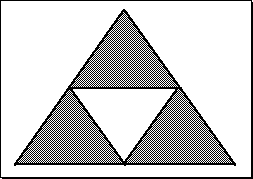Constructing the Sierpinski Triangle.

Iterating the first step.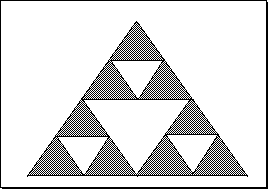Constructing the Sierpinski Triangle.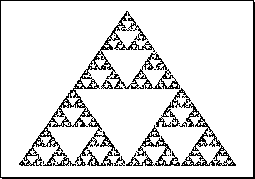The Sierpinski Triangle.

Calculating the dimension... D = log(N)/log(r) = log(3)/log(2) = 1.585. This time we get a value between 1 and 2.

### The dimensionality of a strange attractor

1. The trajectory of a strange attractor cannot intersect with itself. (Why?)
2. Nearby trajectories diverge exponentially. (Why?)
3. But the attractor is bounded to the phase space.
4. The trajectory does not fill the phase space.

A strange attractor is a fractal, and its fractal dimension is less than the dimensions of its phase space.

### Self-similarity

An important (defining) property of a fractal is self-similarity, which refers to an infinite nesting of structure on all scales. Strict self- similarity refers to a characteristic of a form exhibited when a substructure resembles a superstructure in the same form.

### Mandelbrot Set

Found by iterating
zn+1 = zn2 + c.
where z is a complex number. z0=0.
For different values of c, the trajectories either: stay near the origin, or "escape".
The Mandelbrot set is the set of points that are not in the Escape Set.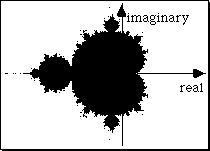The Mandelbrot set. The points in the set are painted black.

The Escape Set differs in rate of escape, graphically depicted with different colors or altitudes ...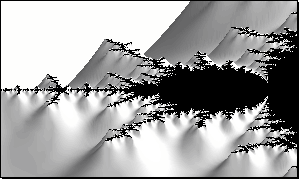Constructed using the computer program "The Beauty of Fractal Lab", by Thomas Eberhardt.### So, what is a fractal?

An irregular geometric object with an infinite nesting of structure at all scales.

### Why do we care about fractals?

• Natural objects are fractals.
• Chaotic trajectories (strange attractors) are fractals.
• Assessing the fractal properties of an observed time series is informative.